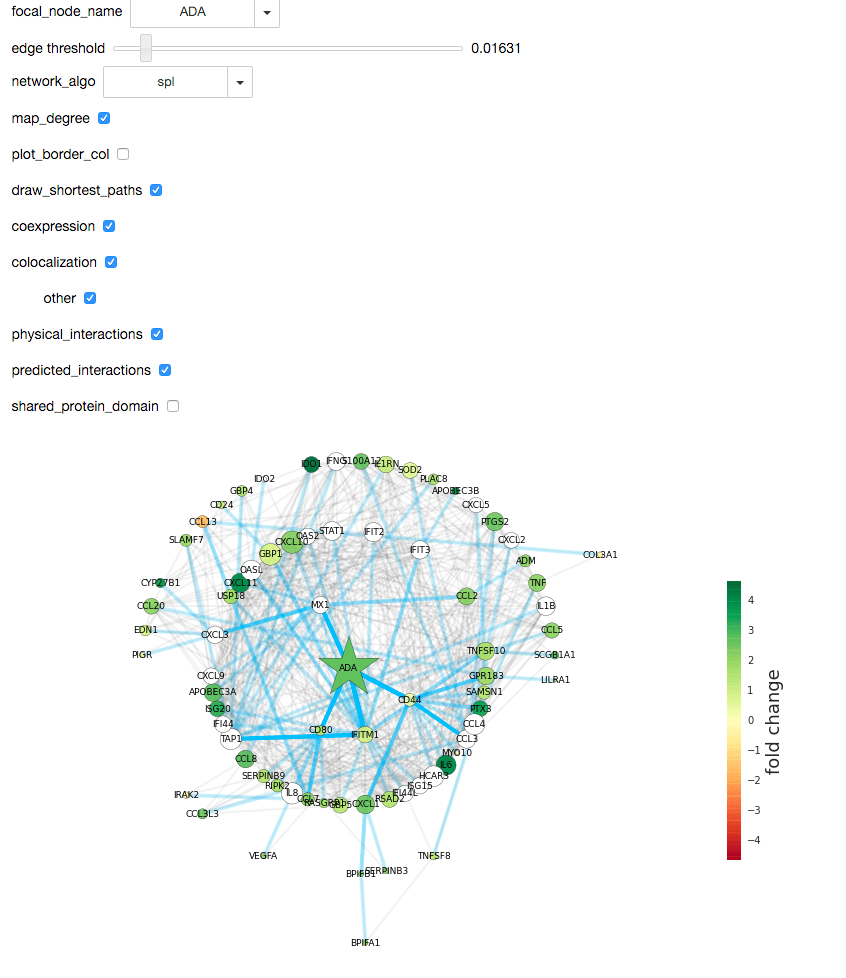## Visualize and analyze differential expression data in a network

In analysis of differential expression data, it is often useful to analyze properties of the local neighborhood of specific genes. I developed a simple interactive tool for this purpose, which takes as input diferential expression data, and gene interaction data (from http://www.genemania.org/). The network is then plotted in an interactive widget, where the node properties, edge properties, and layout can be mapped to different network properties. The interaction type (of the 6 options from genemania) can also be selected.

This notebook will also serve as an example for how to create, modify, visualize and analyze weighted and unweighted gene interaction networks using the highly useful and flexible python package NetworkX (https://networkx.github.io/)

This tool is most useful if you have a reasonably small list of genes (~100) with differential expression data, and want to explore properties of their interconnections and their local neighborhoods.

The interactive ipython notebook version of this post may be accessed here (https://github.com/brinrosenthal/DE_network_visualizer), where you can use the network visualizer with our example data, or insert your own data.

### Import a real network (from this experiment http://www.ncbi.nlm.nih.gov/sites/GDSbrowser?acc=GDS4419)¶

This experiment contains fold change information for genes in an experiment studying ‘alveolar macrophage response to bacterial endotoxin lipopolysaccharide exposure in vivo’. We selected a list of genes from the experiment which had high differential expression, and were enriched for ‘immune response’ and ‘response to external biotic stimulus’ in the gene ontology. This experiment and gene list were selected purely as examples for how to use this tool for an initial exploration of differential expression data.

### Description of options:¶

• focal_node_name: Select gene to focus on (a star will be drawn on this node)
• edge_threshold: Change the number of edges included in the network by moving the edge_threshold slider. Higher values of edge_threshold means fewer edges will be included in the graph (and may improve interpretability). The threshold is applied to the ‘Weight’ column of DE_network, so the less strongly weighted edges are not included as the threshold increases
• network_algo: Select the network algorithm to apply to the graph. Choices are:
• ‘spl’ (shortest path length): Plot the network in a circular tree layout, with the focal gene at the center, with nodes color-coded by log fold-change.
• ‘clustering coefficient’: Plot the network in a circular tree layout, with nodes color-coded by the local clustering coefficient (see https://en.wikipedia.org/wiki/Clustering_coefficient).
• ‘pagerank’: Plot the network in a spring layout, with nodes color-coded by page rank score (see https://en.wikipedia.org/wiki/PageRank for algorithm description)
• ‘community’: Group the nodes in the network into communities, using the Louvain modularity maximization algorithm, which finds groups of nodes optimizing for modularity (a metric which measures the number of edges within communities compared to number of edges between communities, see https://en.wikipedia.org/wiki/Modularity_(networks) for more information). The nodes are then color-coded by these communities, and the total modularity of the partition is printed above the graph (where the maximal value for modularity is 1 which indicates a perfectly modular network so that there are no edges connecting communities). Below the network the average fold-change in each community is shown with box-plots, where the focal node’s community is indicated by a white star, and the colors of the boxes correspond to the colors of the communities above.
• map_degree: Choose whether to map the node degree to node size
• plot_border_col: Choose whether to plot the log fold-change as the node border color
• draw_shortest_paths: If checked, draw the shortest paths between the focal node and all other nodes in blue transparent line. More opaque lines indicate that section of path was traveled more often.
• coexpression, colocalization, other, physical_interactions, predicted_interactions, shared_protein_domain: Select whether to include interactions of these types (types come from GeneMania- http://pages.genemania.org/data/)

## Some examples¶

First let’s look at the graph when ‘spl’ (shortest path length) is selected as the network algo. ADA is the focal node in this case, and it has 4 nearest neighbors (MX1, CD44, FITM1, and CD80). Note that CD44 connects the focal node ADA to many other nodes in the network, as it is an opaque blue line. Also note that there is only one gene with anegative fold change in this gene set (CCL13). The white nodes are genes included by genemania- they are the 20 genes nearest to the input genelist.### Community detection¶

When the network_algo button is switched to ‘community’, the louvain modularity maximization algorithm runs on the network, and partitions the nodes into communities which maximize the modularity. In this case (with CXCL10 as the focal node), the nodes are partitioned into 5 groups, with the three largest groups indicated by red, green, and teal circles. While you can see some support for this grouping by eye, the overall graph modularity is 0.33, which is a relatively low value. This means that although groups were found in the graph, the graph itself is not very modular. As a rule of thumb, very modular graphs have modularities of about 0.5 or 0.6.Below the graph, there is a panel showing the average fold change for the nodes in this community. Since most of the nodes in the input gene list have positive fold changes here, all communities also have positive average fold changes. Were the input gene list to have fewer large fold changes, this would enable you to see if a particular grouping of nodes had significantly higher (or lower) levels of differential expression than alternative groupings.« Return